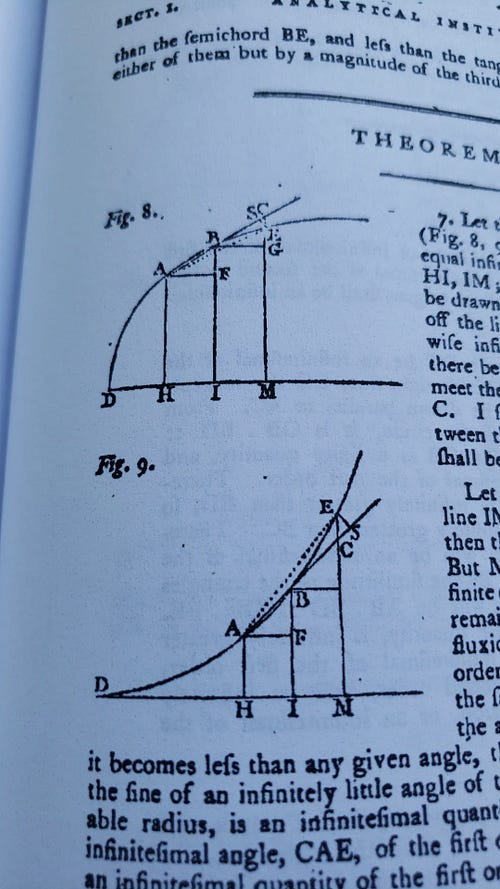# Maria Agnesi and the Second Derivative

I’m currently reading selected parts of “Analytical Institutions”, the 1801 edition of John Colson’s translation of Maria Gaetana Agnesi’s 1748 text.

Near the beginning of her second book, she presents the following theorem. Consider the diagrams:Figures 8 and 9, Book II, Section I, Theorem II

In each diagram, H, I, and M are points along the x-axis and are equally spaced. A, B, and E are points along “any curve whatever”, such that the three segments (HA, IB, and ME) are perpendicular to the x-axis.

We draw the secant line that contains A and B, then we place C as the intersection of AB and ME (extending ME as needed).

What interests us in this theorem is the length of the segment CE, but let’s step back a second.

The slope of AB, the secant line, approximates the slope of the line tangent to the curve at A: As Δx decreases (that is, as AH, BI, and EM get closer together), this approximation improves.

For instance, consider the function f(x= x².

Using Δx = .1: f(1) = 1 and f(1.1) = 1.21. We can calculate the slope of the line through (1, 1) and (1.1, 1.21) as 2.1.

Using Δx = .01: the slope of the line through (1, 1) and (1.01, 1.0201) is 2.01. The slope of the line through (1, 1) and (1.001, 1.002001) is 2.001. If we repeat this process forever, the slope will get increasingly close to 2.

Meanwhile, since the derivative f’(x) = 2x, we can calculate the exact slope of the tangent at x=1 to be 2.

Okay, so, that’s something that’s covered in basic high school calculus:

The slope of the line tangent to a function is equal to its first derivative.

That’s not what Theorem II is about. Instead, Agnesi looks at CE, that little segment that separates the secant line from the curve.

She writes, “I say, that the intercepted line CE, between the curve and the chord AB produced, shall be an infinitesimal of the second order.” This is the theorem that she proceeds to prove.

If her theorem is correct, there must be some relationship between the length of EC (as an approximation) and the second derivative of the function.

Given f(x) above, f’’(x) = 2. What is the length of EC?

We can write a formula for the secant line. Given Δx = .1, the secant line is y = 2.01 (x-1) + 1. At = 1.2 (adding 2Δx), that line contains the point (1.2, 1.42). Meanwhile, f(1.2) = 1.44, so our secant line is off by .02.

Since this represents a second order derivative, what happens when we divide that gap by Δx²? We get (.02)/(.1)² = 2.

The length of CE divided by Δx² is an approximation for the second derivative.

This is the ramification, in modern terms, of Agnesi’s theorem.

Let’s try a more complicated example. Let g(x) = x⁴ + 4x³Then g’(x) = 4x³ + 12x² and g’’(x) = 12x² + 24x.

Evaluate this at 3 with Δx = .01. The exact value of the second derivative is 180; the value we get from |CE|/Δx² is 180.961. Not perfect, but close enough for estimation purposes.

Using Δx = .00001 gives |CE|/Δx² = 180.001, which is much better.

Furthermore, Agnesi notes that EC will have a negative value if C is greater than E, and positive if E is greater than C. This was a significant a-ha! for me.

I have struggled with understanding what the second derivative means. The first derivative represents how quickly a function’s values are changing, but that means the second derivative represents how quickly that change is changing.

A poor understanding here leads to me having to simply memorize the concavity rules (if the second derivative is positive, the function is concave upwards). I usually think of x², which I know is always concave upwards and which I know has a second derivative of 2. But that feels like a superficial understanding.

Seeing this visual explanation helps me make sense of it now, in a way that makes so much more sense. The segment EC represents the aggregate error between the secant line (an estimate) and the value of the function. If EC is positive (that is, E is greater than C, as in Agnesi’s figure 9), the estimate low-balls because the function is growing more quickly, and hence the graph’s curve will be concave upwards.

If EC is negative (as in figure 8), the estimate is too high because the function is growing less quickly, and the curve will be concave downwards.

In other words, the secant line is growing at a steady rate: The sign of the error indicates which direction the function is curving away from that secant line.

If you’d like to see this in action (and play around with it), here is a link to a Desmos file. Just edit the function as you please, play with the two sliders, and compare the last two values.Clio Corvid